Browse by Topic

# Quantum Computation: Wolfram Language Meets Amazon Braket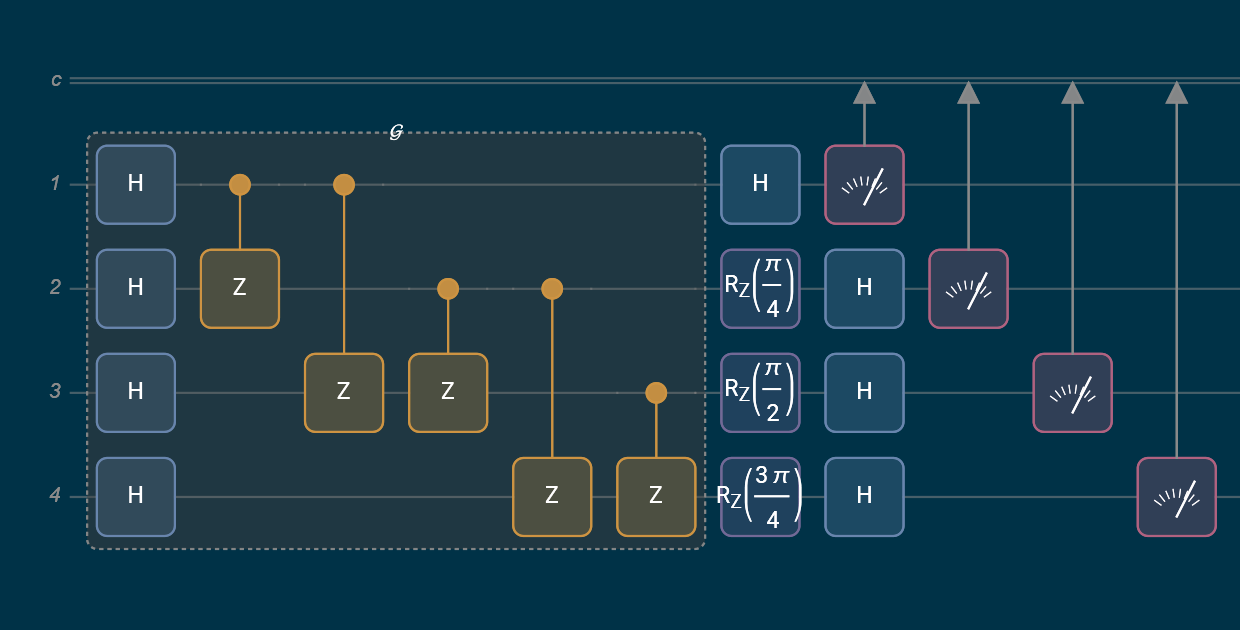The collaboration between Wolfram Language and Amazon Braket is propelling quantum computation research to unprecedented levels. By combining Amazon Braket’s advanced quantum capabilities and Wolfram’s expansive knowledgebase and accessible symbolic language, users can now push the boundaries of quantum research.

Amazon Braket is a quantum computing service on Amazon Web Services (AWS) with the mission of accelerating the development of quantum computing applications. With Amazon Braket, users can explore various types of quantum processing units (QPUs), each with its own physical implementation. This provides a unique opportunity to compare different technologies side by side. Additionally, Amazon Braket is focused on envisioning how quantum computing will eventually fit into a cloud-based IT infrastructure, working alongside other computational resources. While current workloads are experimental, it is crucial for the field to begin addressing complex operational issues such as usability, security, resource management and more.

The Wolfram Quantum Team has designed a paclet, called the Wolfram Quantum Framework and available from the Wolfram Language Paclet Repository, to aid in quantum research. The Wolfram Quantum Framework is a software tool that enables the development and simulation of quantum algorithms using Wolfram Language. Its key features include a library of pre-built quantum functions (such as those for quantum Fourier transforms or Grover’s search algorithm), integration with the Wolfram Cloud and the ability to simulate quantum systems symbolically as well as numerically.

The combination of Wolfram Language and quantum computation offers an unparalleled opportunity to perform both complex calculations and quantum tasks on QPUs with ease. With the addition of Amazon Braket, this powerful duo can take on even the most challenging computational problems. So let’s jump in and explore the Wolfram Quantum Framework and connect it with Amazon Braket through the AWS service.

## The Wolfram Quantum Framework in Action

To utilize the Framework paclet, use the following click-to-copy code to install and load it: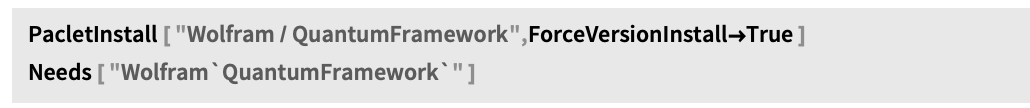Let’s look at a few examples. A Grover circuit can be used to find solutions of a Boolean function: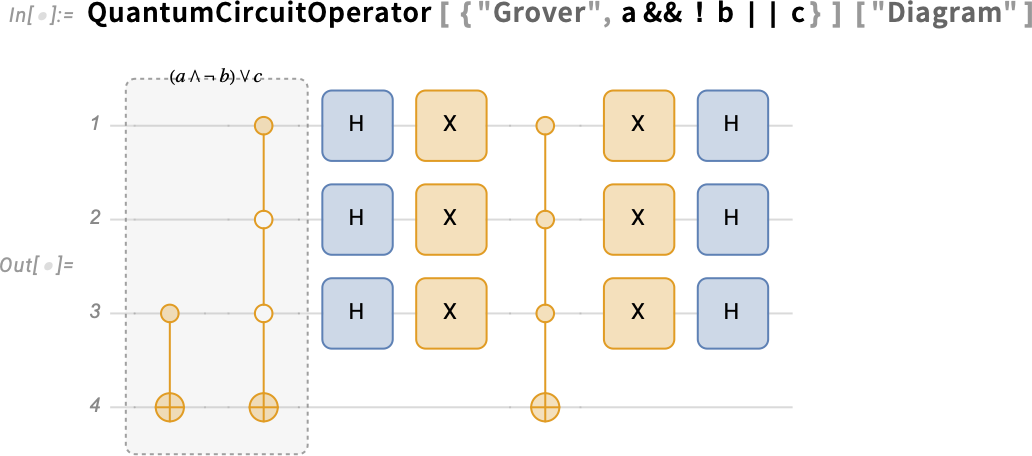A quantum circuit for a Fourier transform of 4-qubits: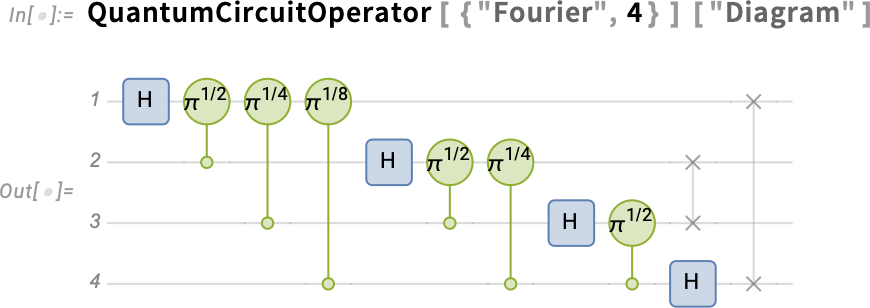A multiplexer circuit, also called a uniformly controlled gate: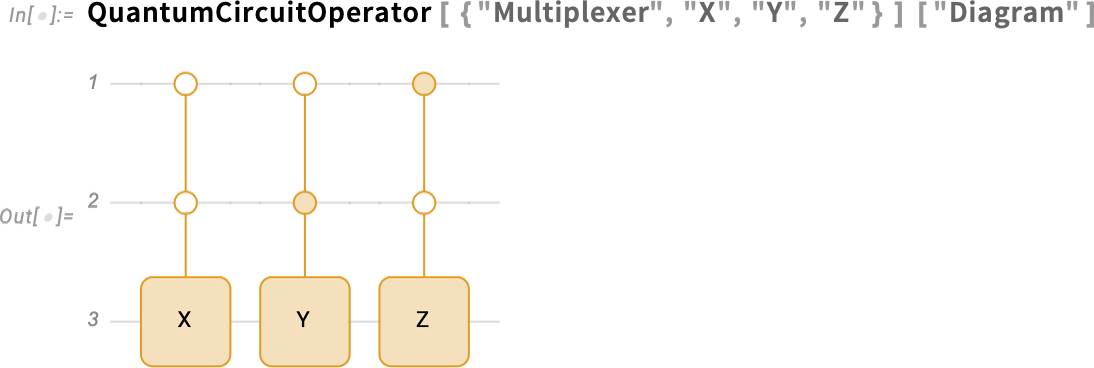With the seamless integration between symbolic language and quantum computation provided in the Framework, users can perform a variety of quantum tasks, including the generation of highly entangled graph states, which are also known as cluster states. These graph states are essential for measurement-based quantum computing. Let’s delve into this specific example for further insights.

Generate a graph with four vertices and five edges: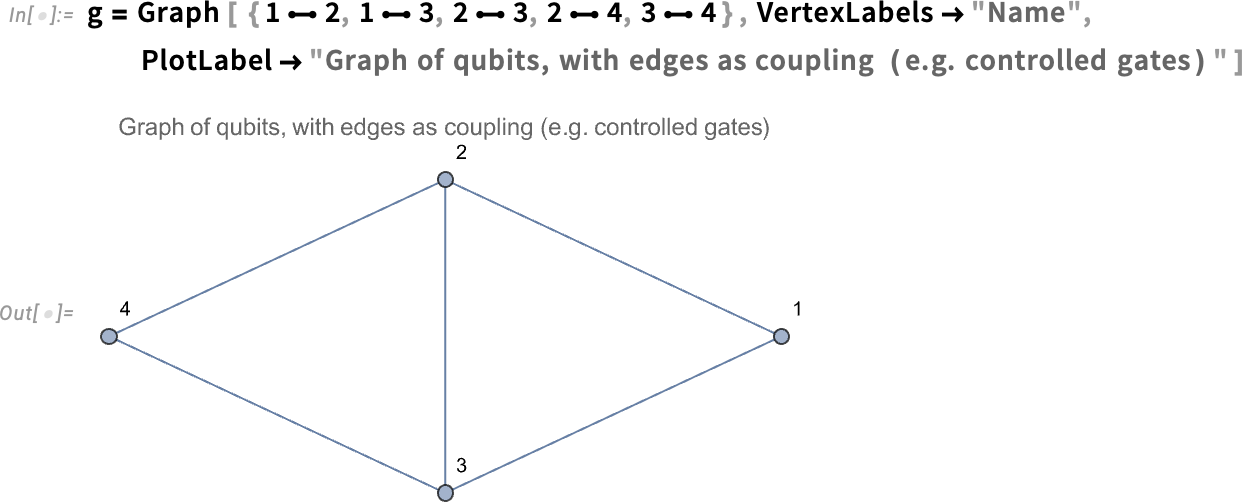Generate the corresponding quantum state of the produced graph: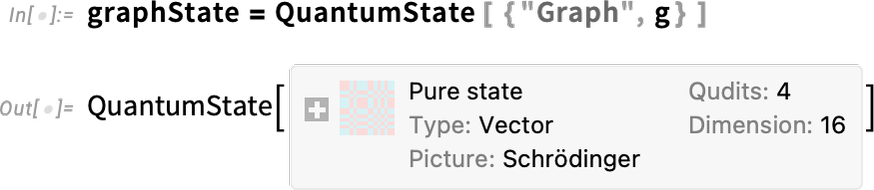It is worth noting the output is a quantum object with some interesting features, such as its type and dimensions.

We will then return the formula of the previous state in the computational basis: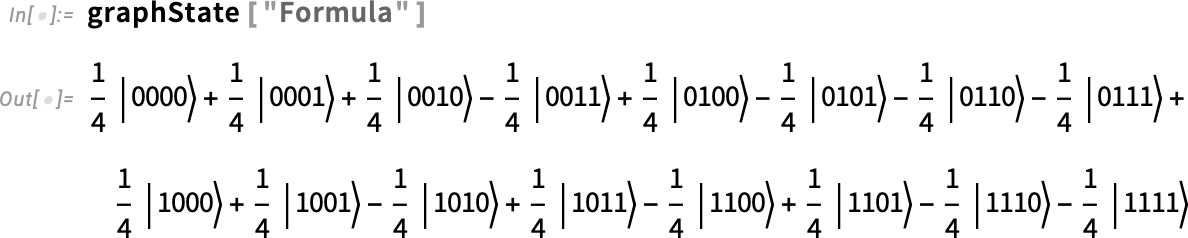Test if the previous graph state is entangled: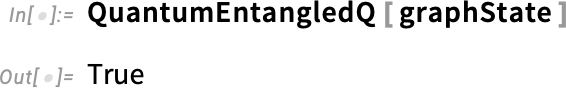Test if qubits 1 and 4 are entangled (after tracing out qubits 2 and 3):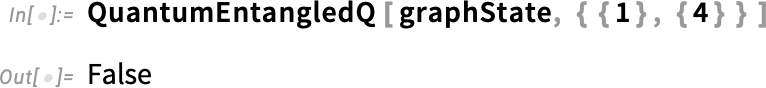Repeat the test, but this time, explicitly trace out qubits 2 and 3 and test the entanglement: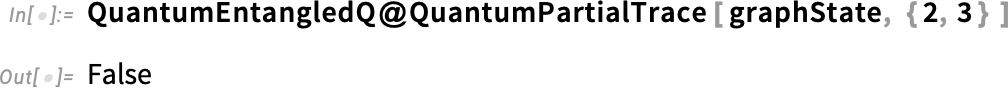In fact, it is interesting to note that the only qubits that are entangled are those connected via an edge: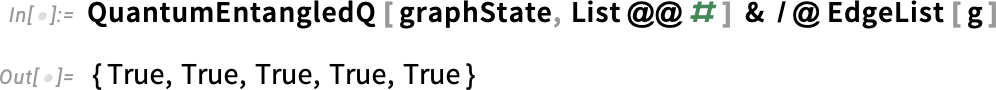Calculate QuantumEntanglementMonotone using different measures: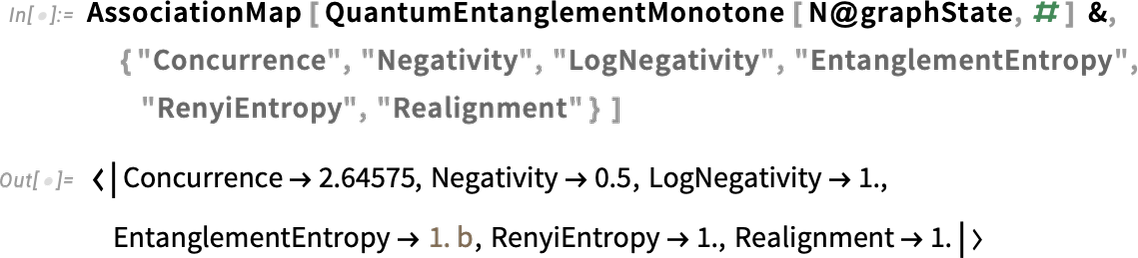Given a vertex of the graph, we can find the list of vertices adjacent to it: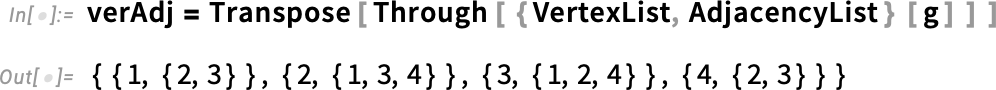Based on the generated list of vertices and their adjacencies, we will need to find the corresponding stabilizer. This can be obtained by applying Pauli-X on vertices and Pauli-Z on adjacencies: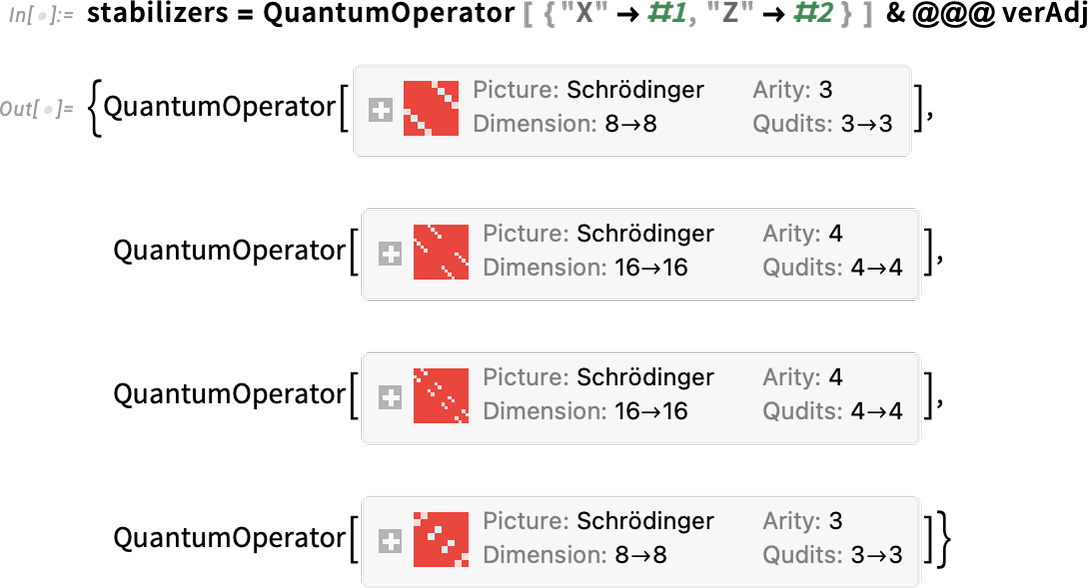Apply this list of stabilizers and show that the result (i.e. the graph state being transformed by a stabilizer) is still the same as the original state; this is why it is called a stabilizer: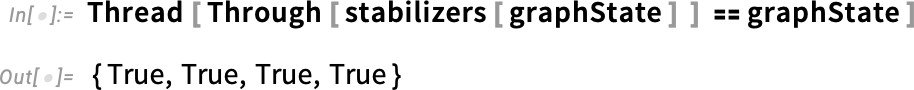Now let’s see how to generate the graph state using a quantum circuit. In other words, the following will design a quantum circuit that transforms a register state into a desired graph state.

To do so, one can use the built-in graph circuit in the Framework. This will produce a corresponding circuit for generating the same graph state: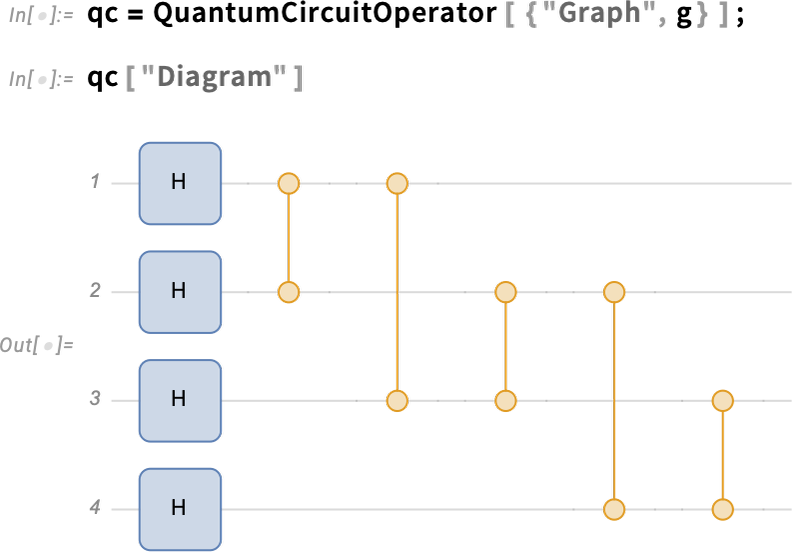Test the result is the same as expected (i.e. the circuit acting on the register state produces the desired graph state):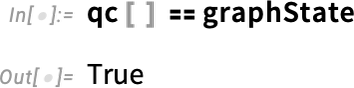Based on the previous circuit, we can see the "TensorNetwork" of the circuit, which also uses indices as graph vertices and tensors as cliques: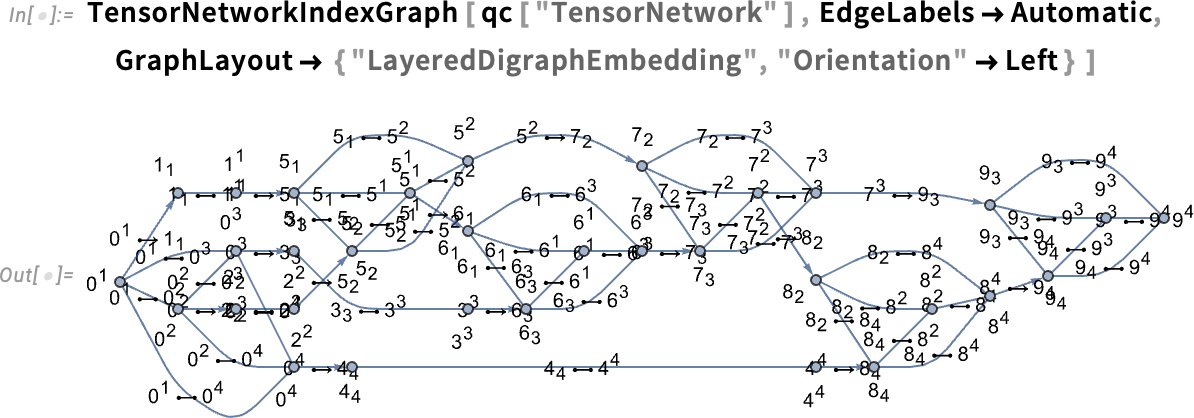Additionally, one can create the corresponding multiway graph of the circuit—where branching happens with entanglement creation (i.e. in each branch, there is not entanglement and the corresponding quantum state is fully separable):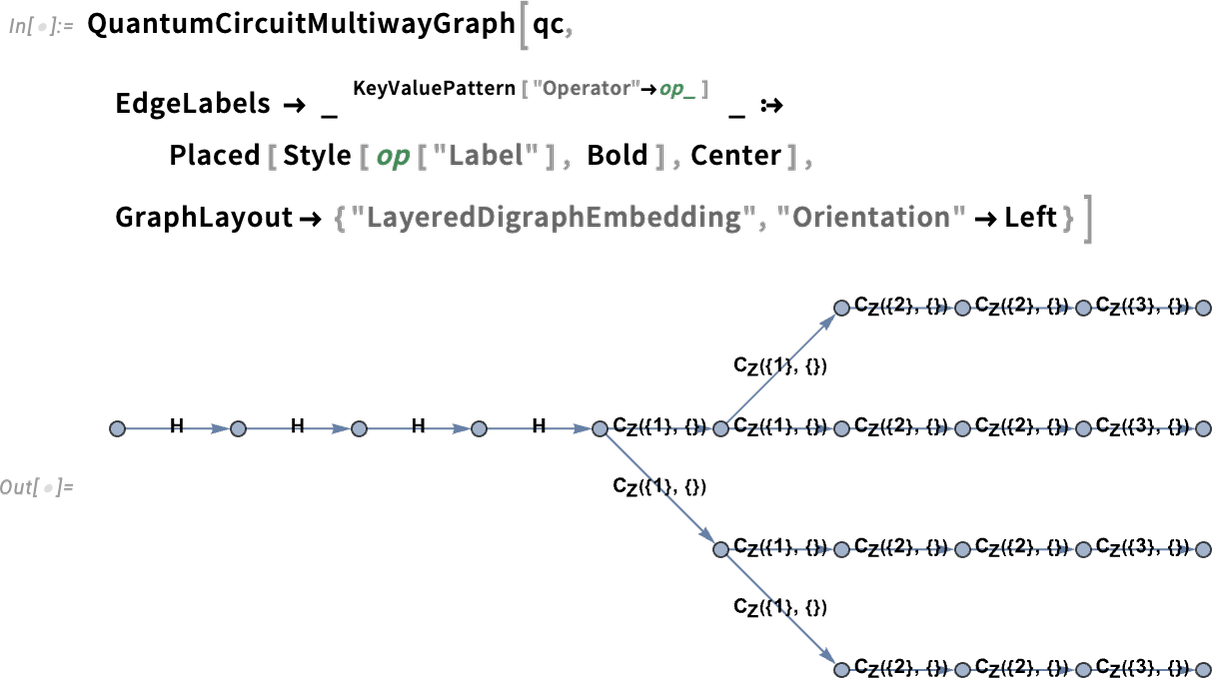Given the circuit for generating a graph state, let’s now measure each qubit in a specific direction on the xy plane. For qubit 1, the measurement will be done along the x axis (corresponding to the Pauli-X measurement) and then for the next qubits, we will rotate the basis of measurement by π/4 around the z axis: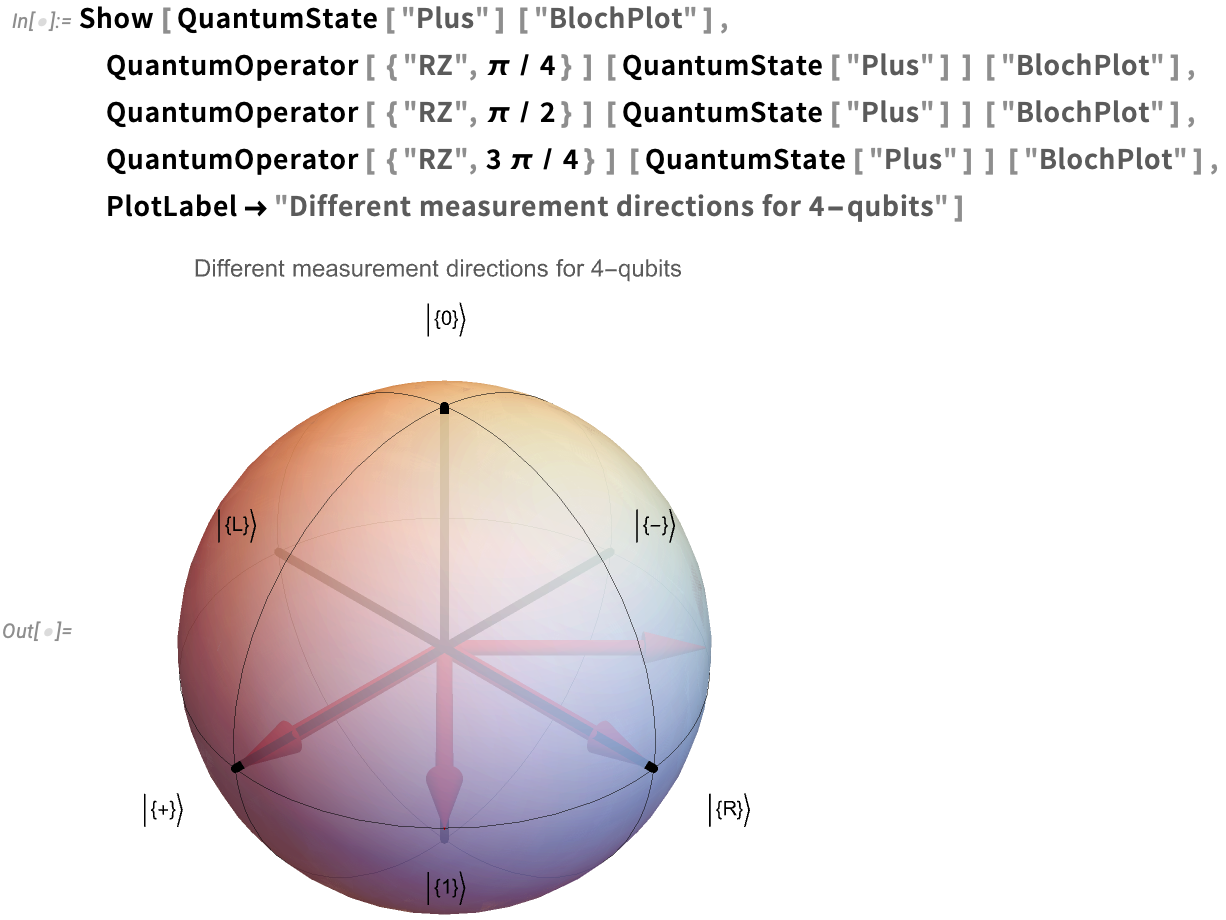From a computational basis, we can apply Hadamard matrices, which marks the measurement of qubits along the x axis (i.e. the Pauli-X measurement). Then we apply the z rotation gates to rotate the measurement basis within the xy plane by a given angle: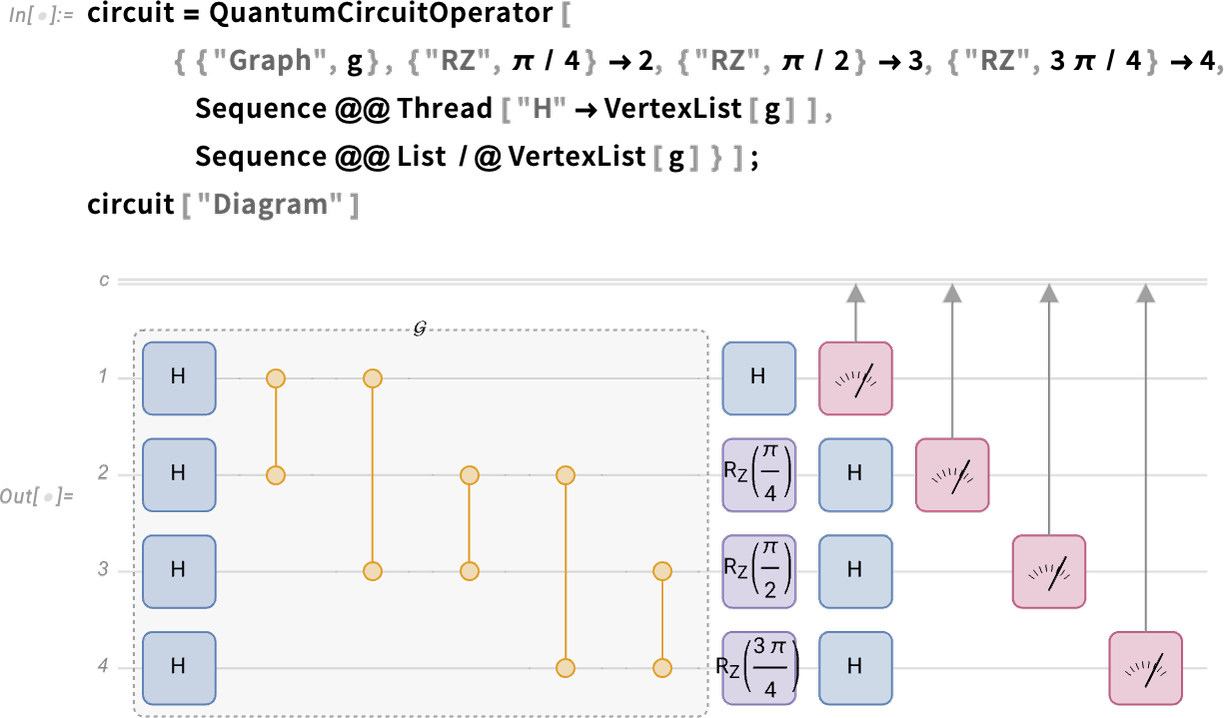Now let’s find out what quantum theory predicts to be the probability distribution one gets from the previous circuit. In the Framework, whenever there is a quantum measurement, the output will be a quantum measurement object: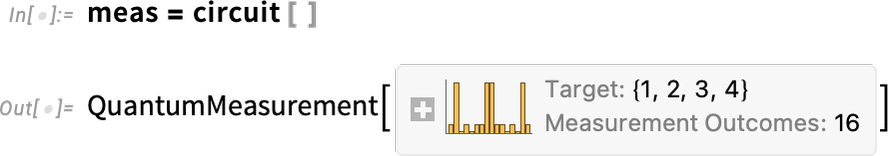There are many interesting features that one can get from a quantum measurement object. For example, the probabilities are calculated exactly using statistical functionalities in Wolfram Language: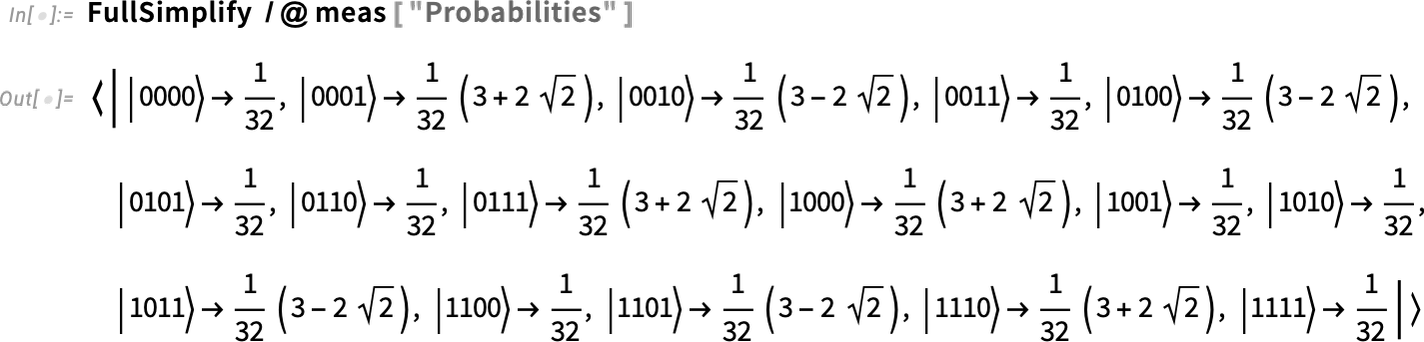Or one can directly ask for the corresponding plot: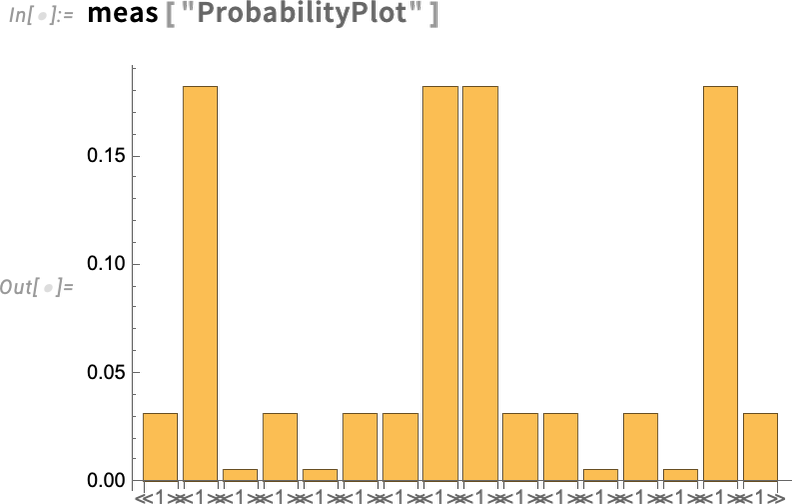Additionally, one can simulate measurements for a given number of shots. This means repeating each measurement and counting what outcome one gets in each run (which is practically one realization of the expected result from a noiseless QPU):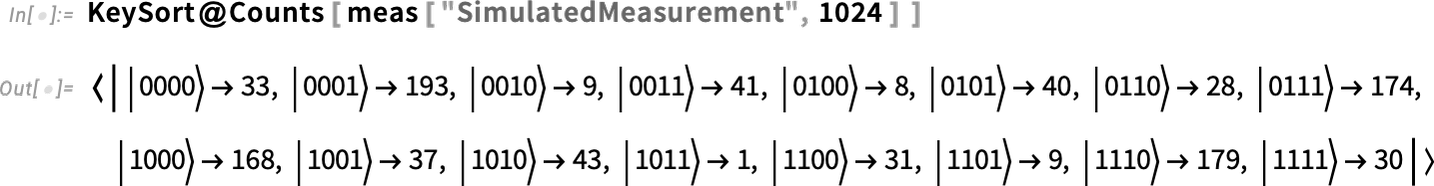## Amazon Braket and Wolfram Language Combined

Let’s jump in and explore how to utilize the power of Amazon Braket’s quantum computing resources using Wolfram Language.

Connect to AWS using your credentials (using your access key ID and secret access key) so the following code will evaluate correctly in your own notebook: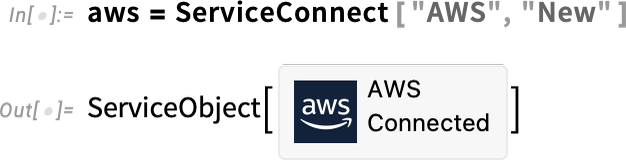For more details on this step, refer to the Wolfram documentation page “Authenticate with Amazon Web Services.”

Execute S3 on AWS (if you do not have an account set up with S3, you will need to create one):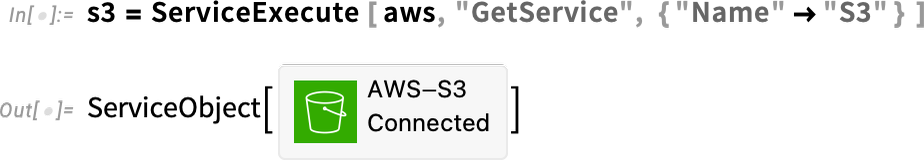Execute Amazon Braket on AWS: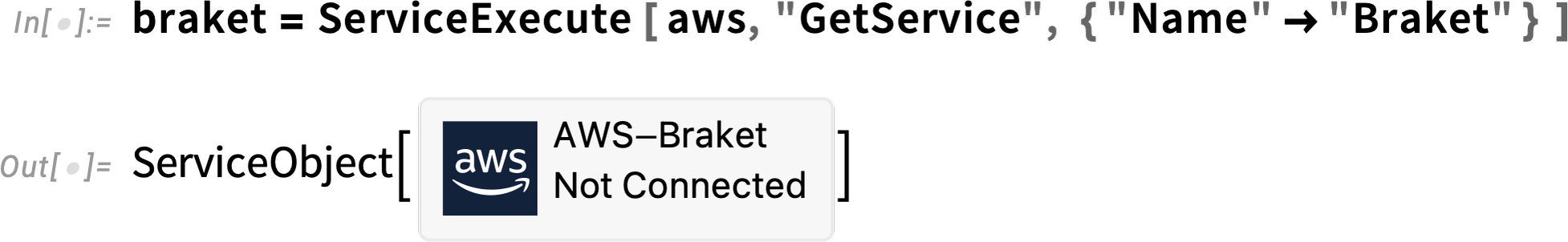At this point, you will be connected to Amazon Braket and able to run quantum algorithms on both simulators and QPUs. You will then need to fetch an available device: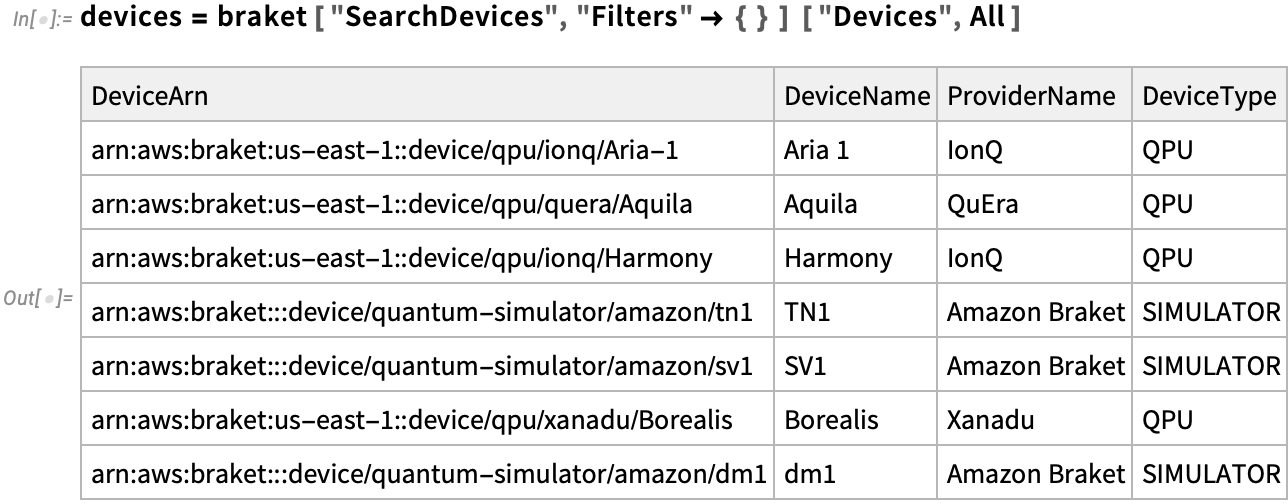In this example, we will use the dataset for an existing QPU from IonQ Harmony and a simulator named SV1 from Amazon: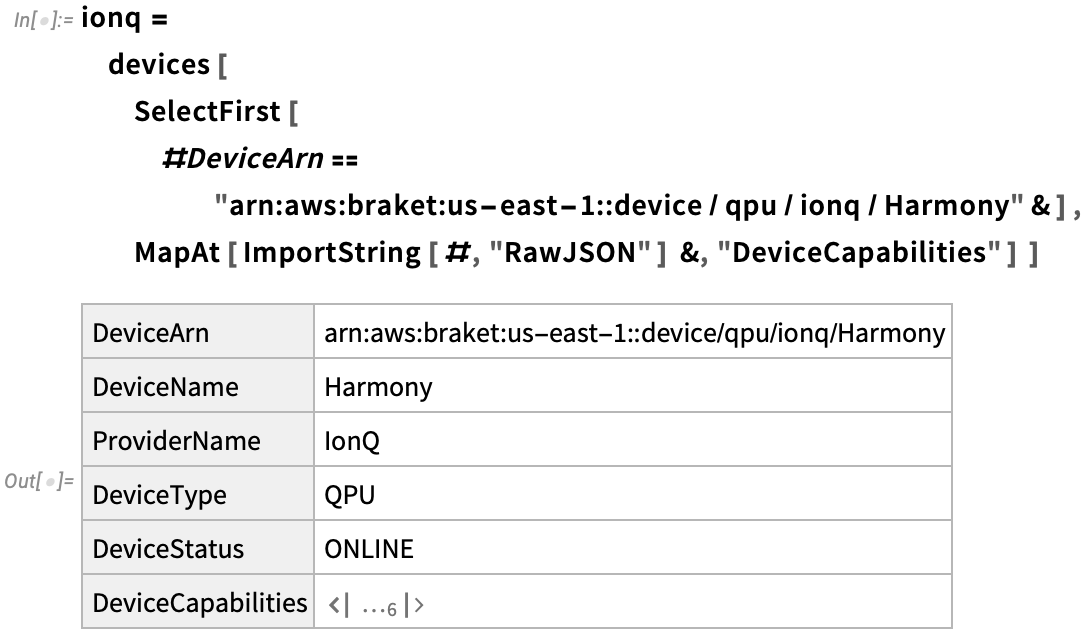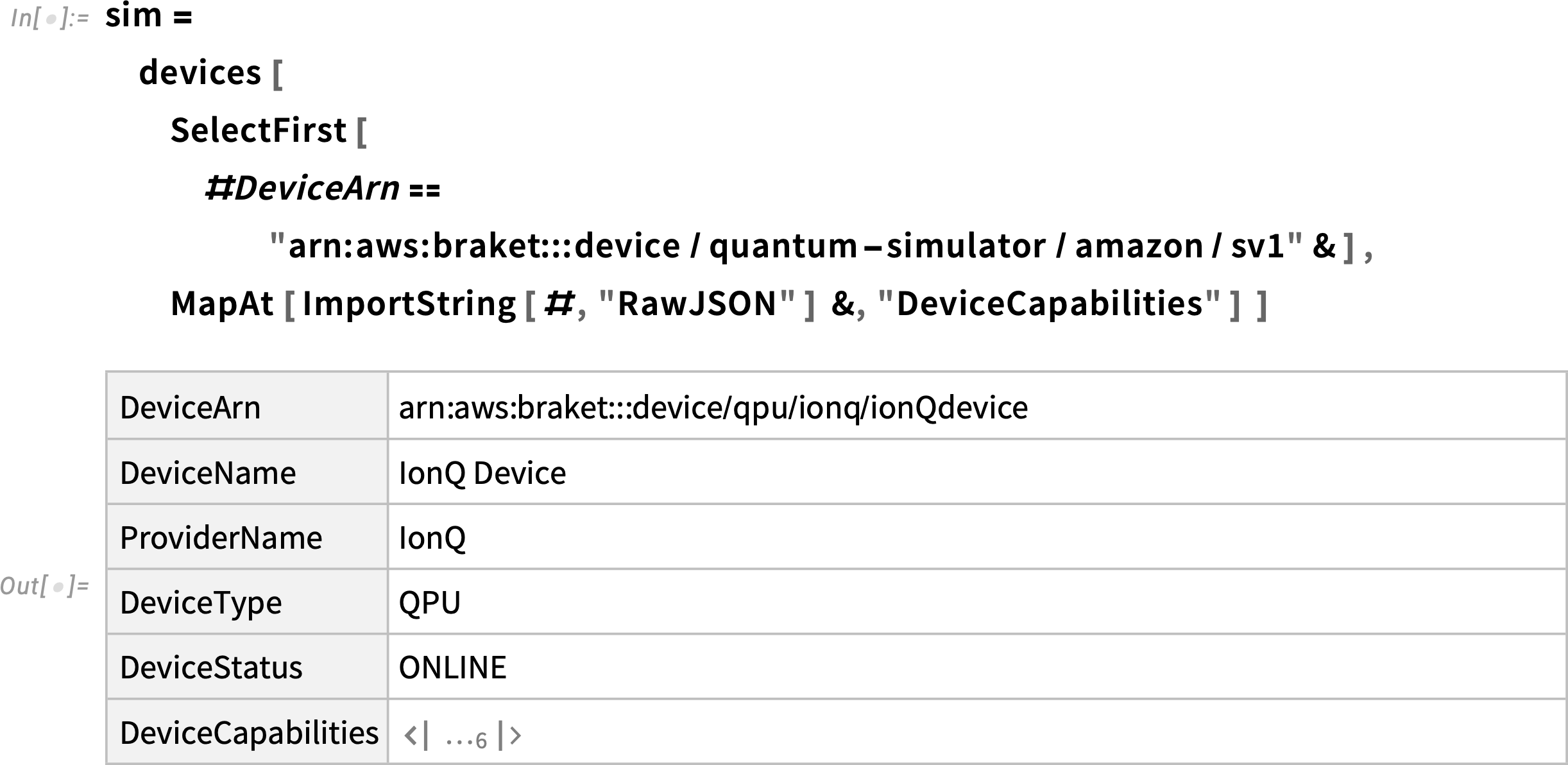Next, we will use the generated OpenQASM code to integrate with the SV1 simulator. Using "AWSBraket" as a provider, we can then generate the corresponding OpenQASM3 code: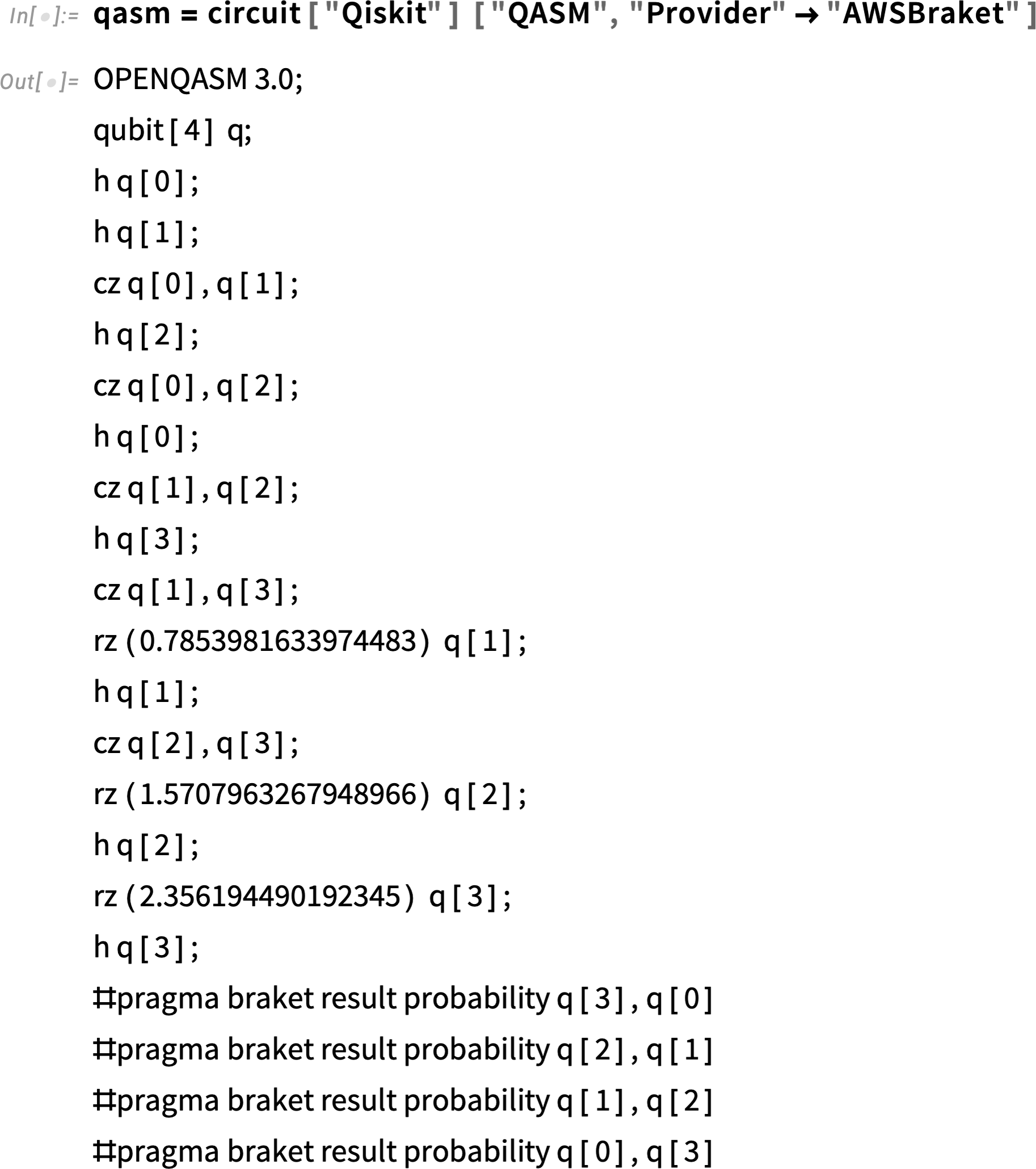Now we can send the query to the Amazon simulator and our personal "OutputS3Bucket":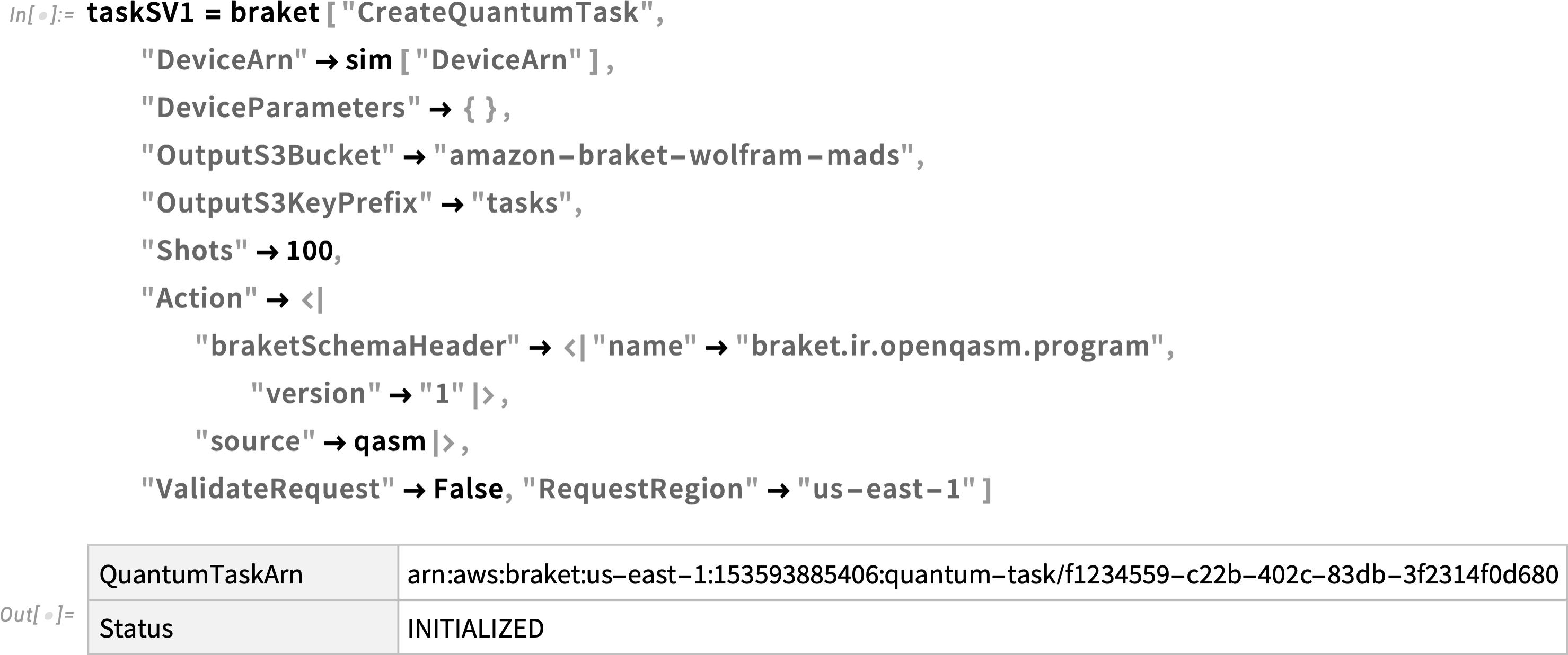Then we will generate OpenQASM code for the IonQ device: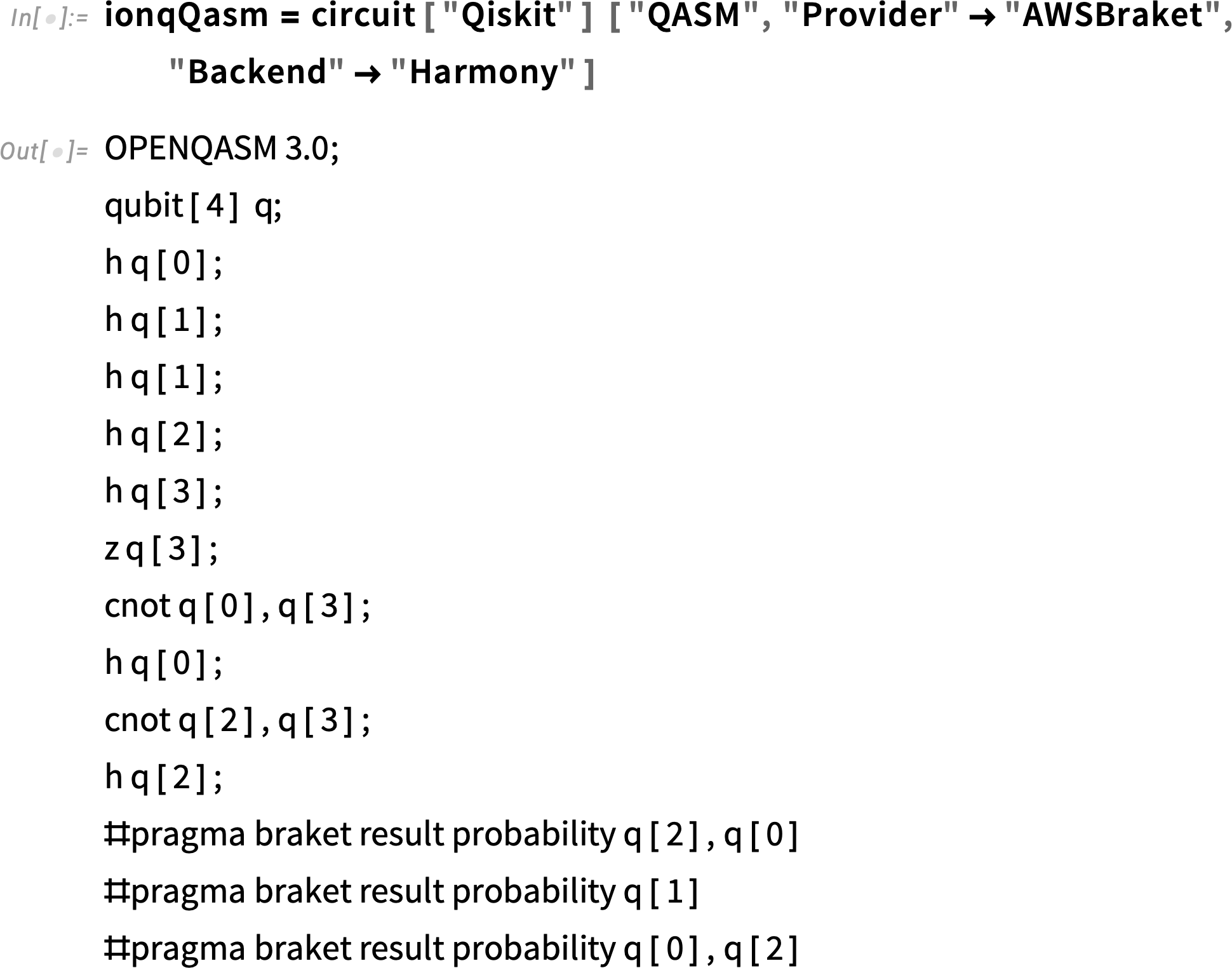Using this OpenQASM code, we can send a query to the IonQ Harmony we picked before: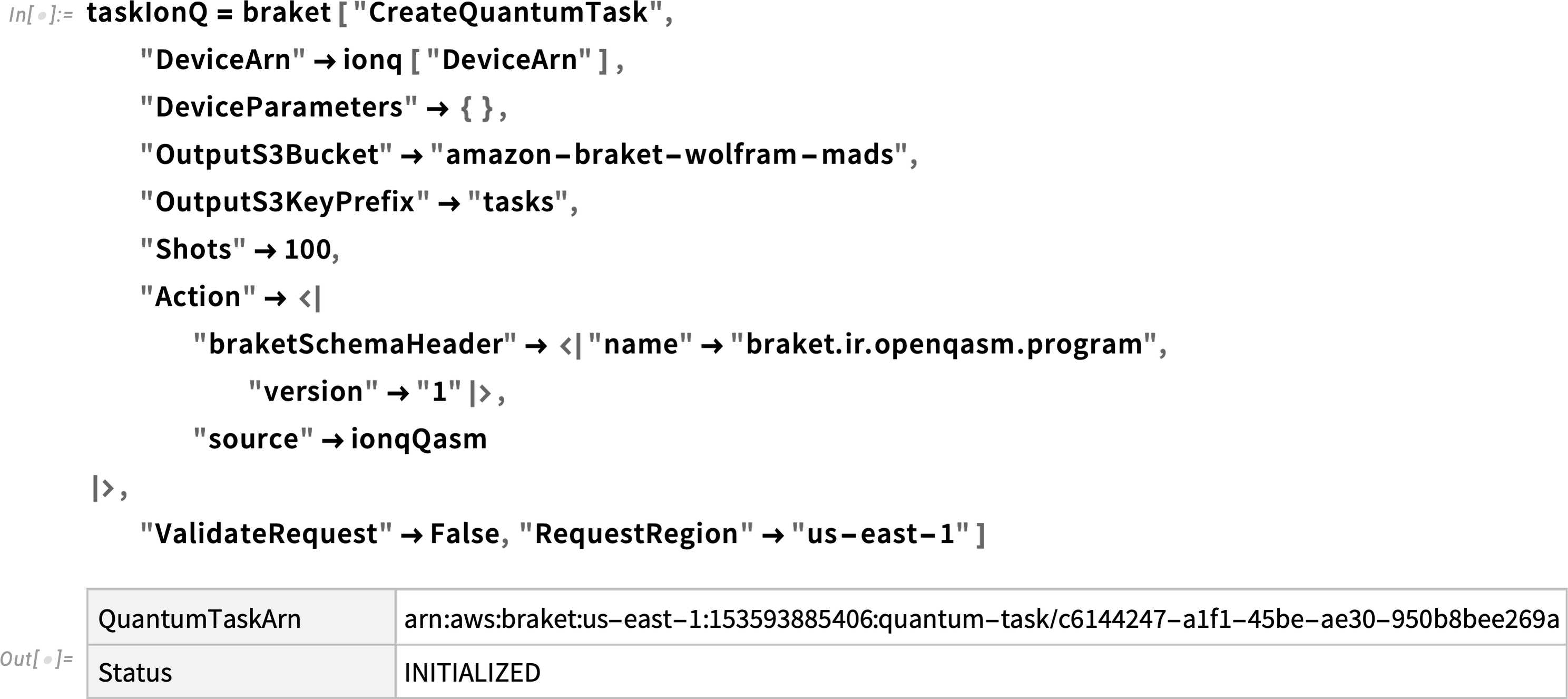We will then request detailed information on our task. By reviewing the generated output, we can see the status of the SV1 task is “COMPLETED”: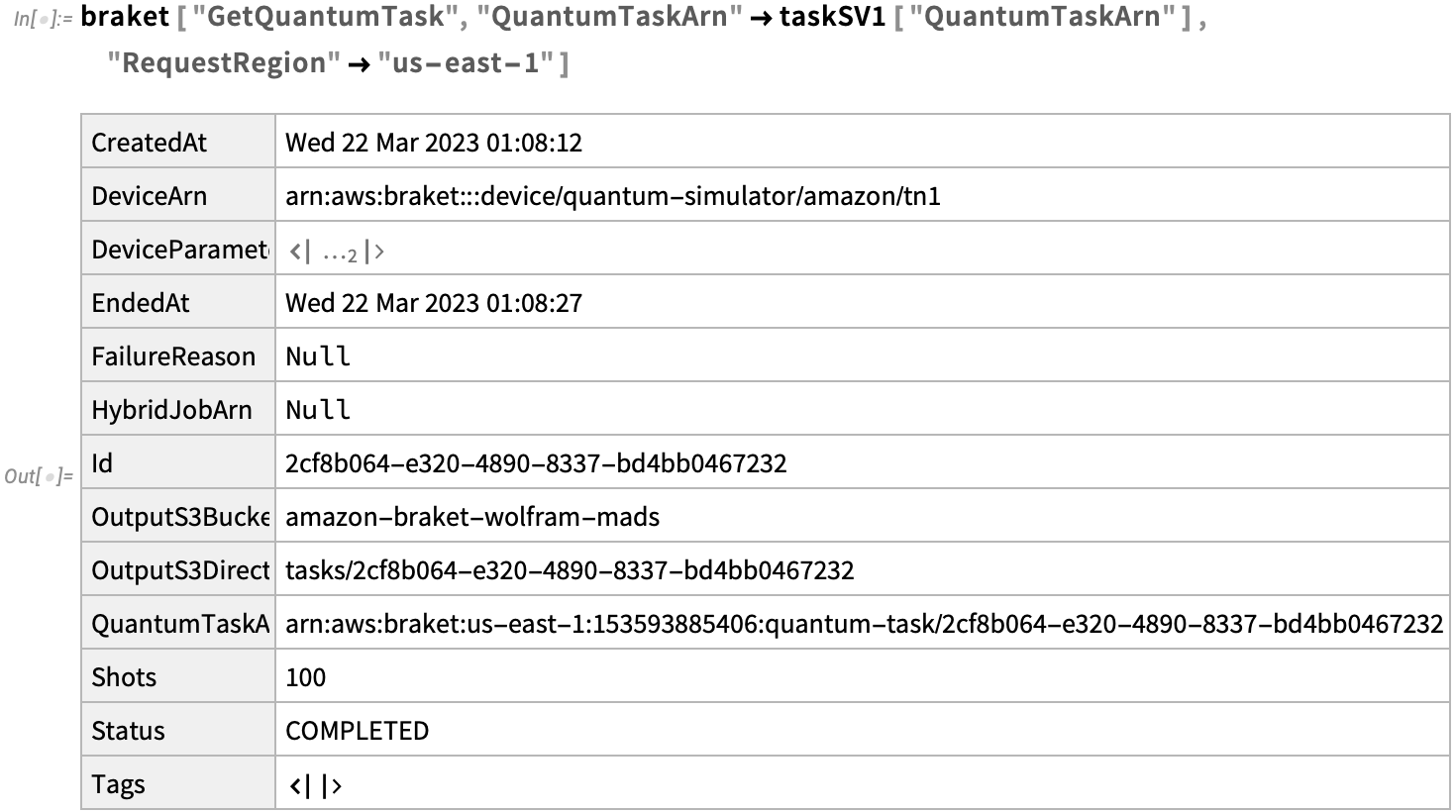We will do the same for the IonQ QPU, but it will take a little longer. By reviewing the generated output, we can see the status of this task is “QUEUED”: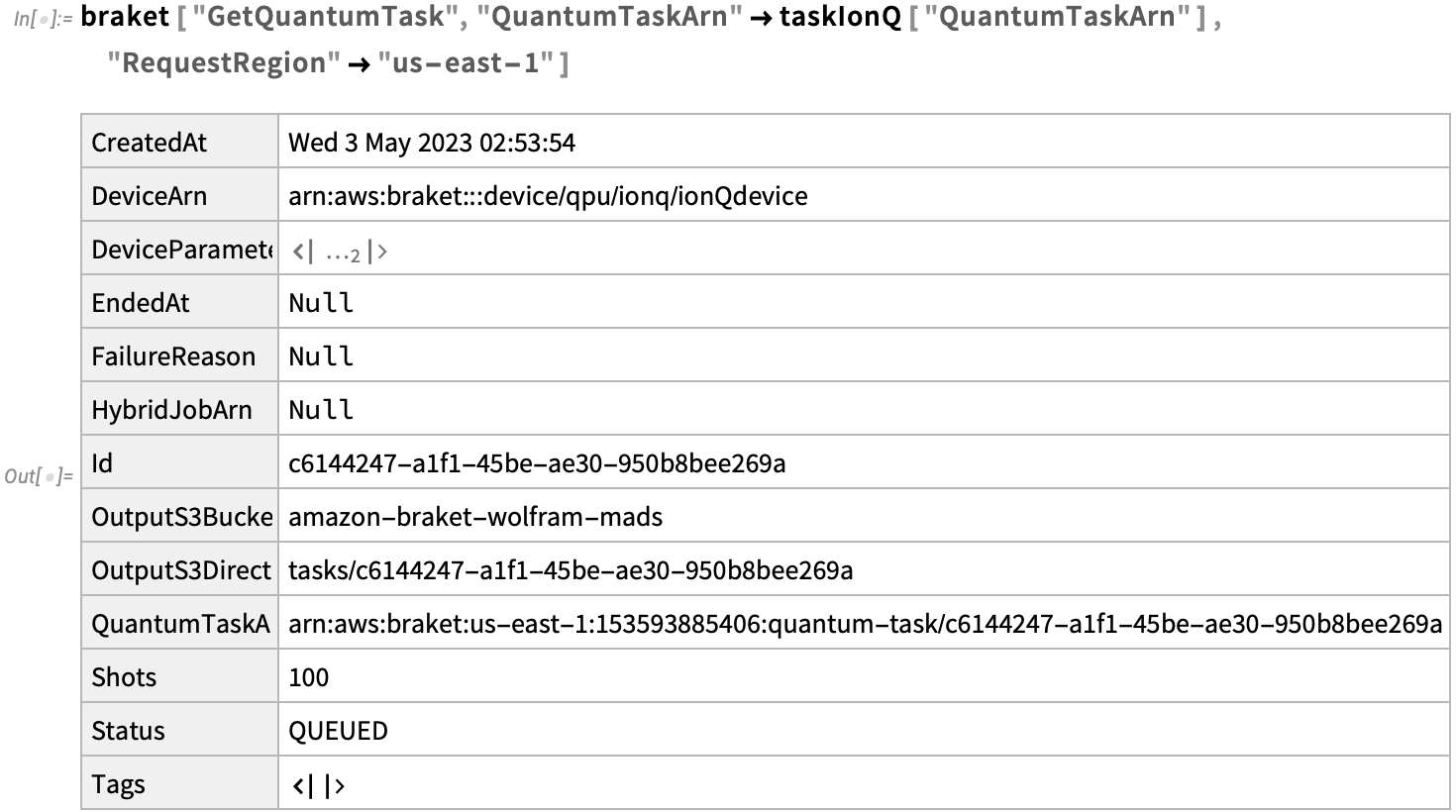The results of the requested updates are saved in S3. We can find these by locating their directories: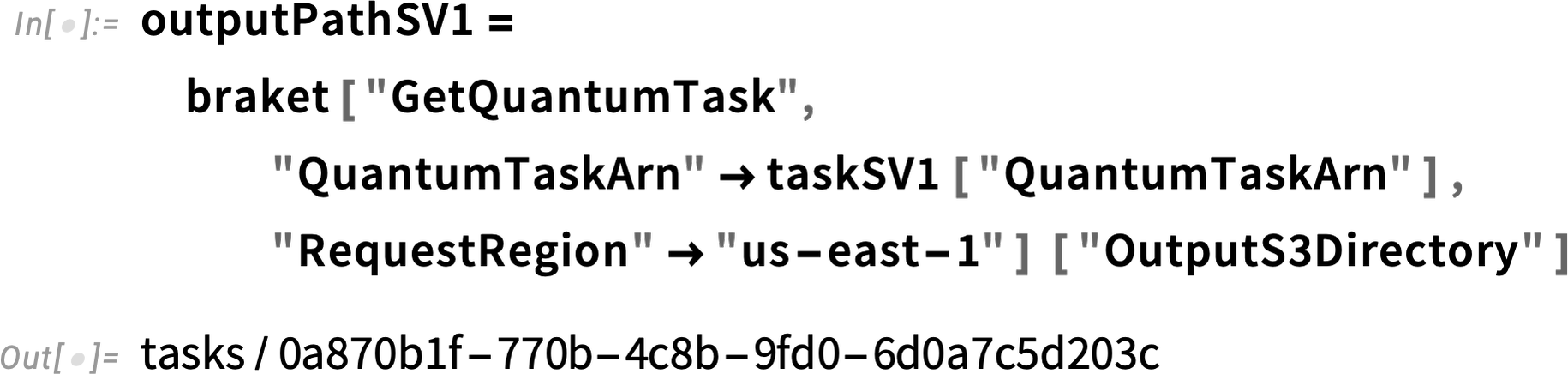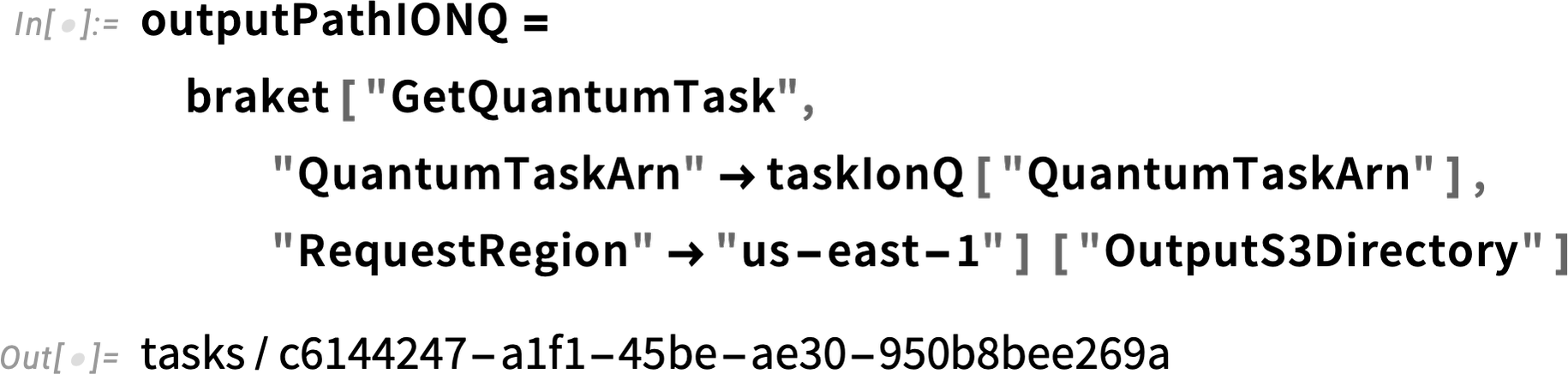Given the previous directories, the measurement results can be obtained as follows. It is worth noting that the SV1 directory returns results as counts per "measurements", while IonQ returns results as "measurementProbabilities". Later on, we will also calculate SV1 results as "measurementProbabilities" for the sake of comparison: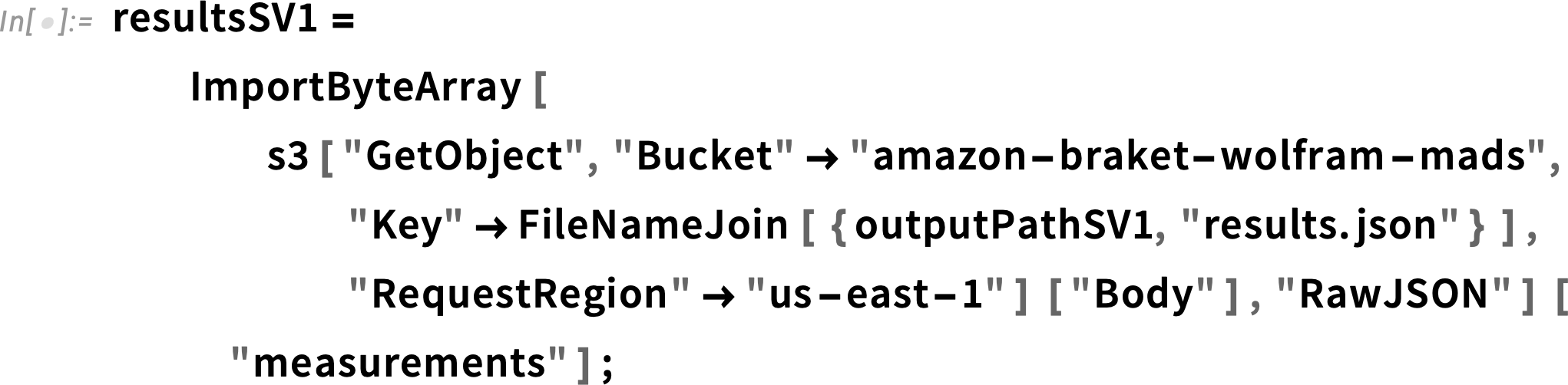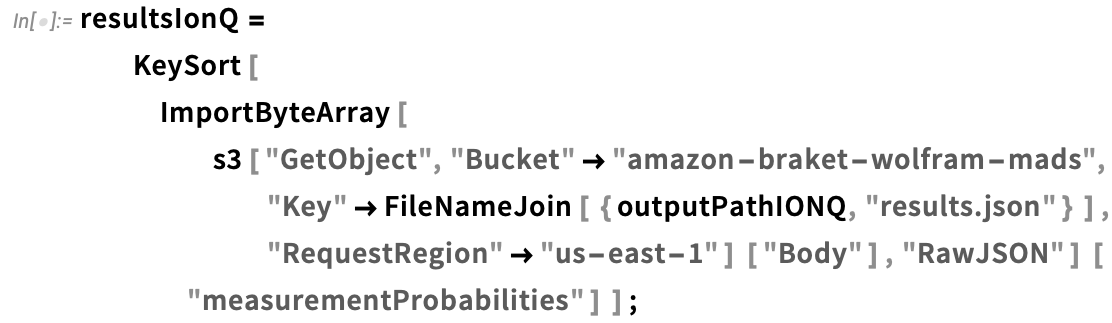Based on the generated results for both SV1 and IonQ, we can then calculate the probabilities: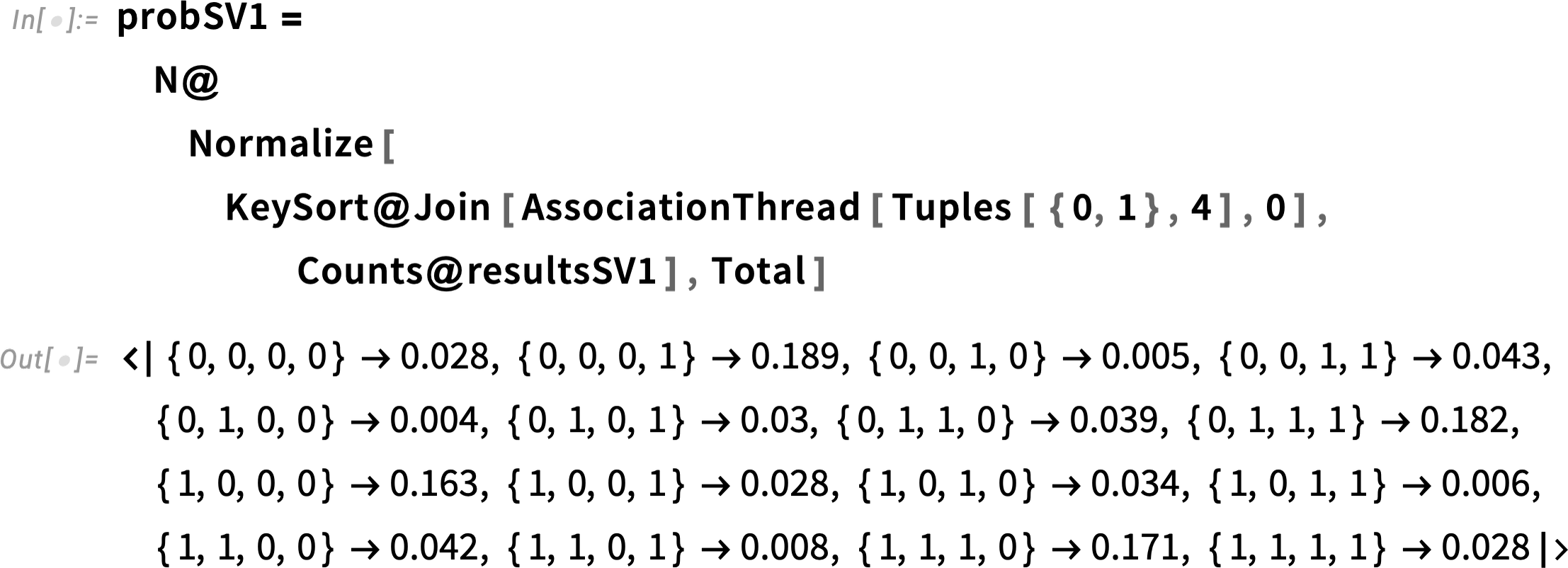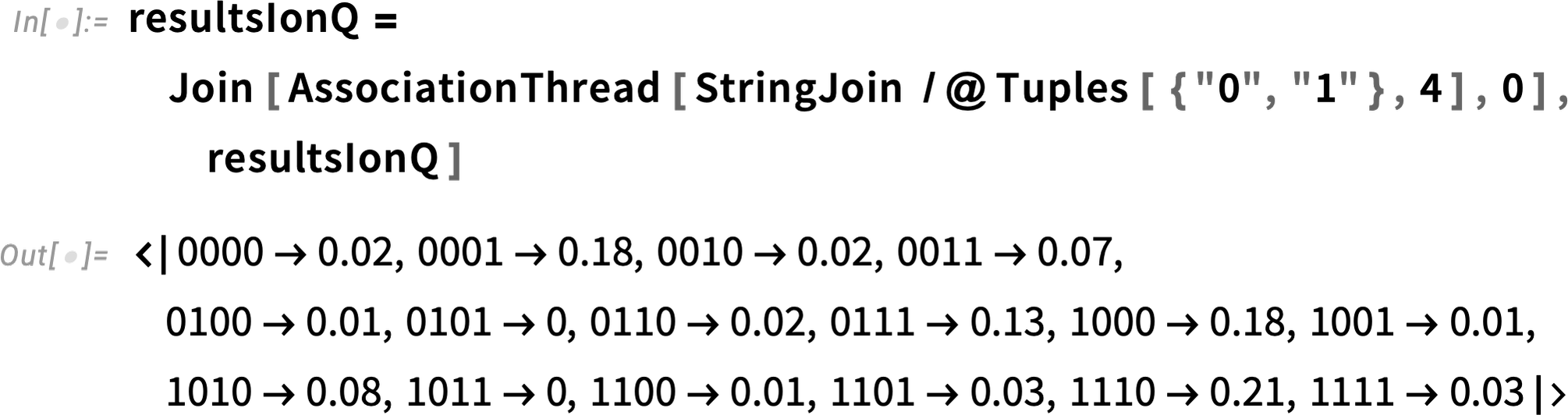We can then chart results for the SV1 simulator (100 shots), IonQ QPU (100 shots) and the Wolfram Quantum Framework (exact probabilities as predicted by quantum theory):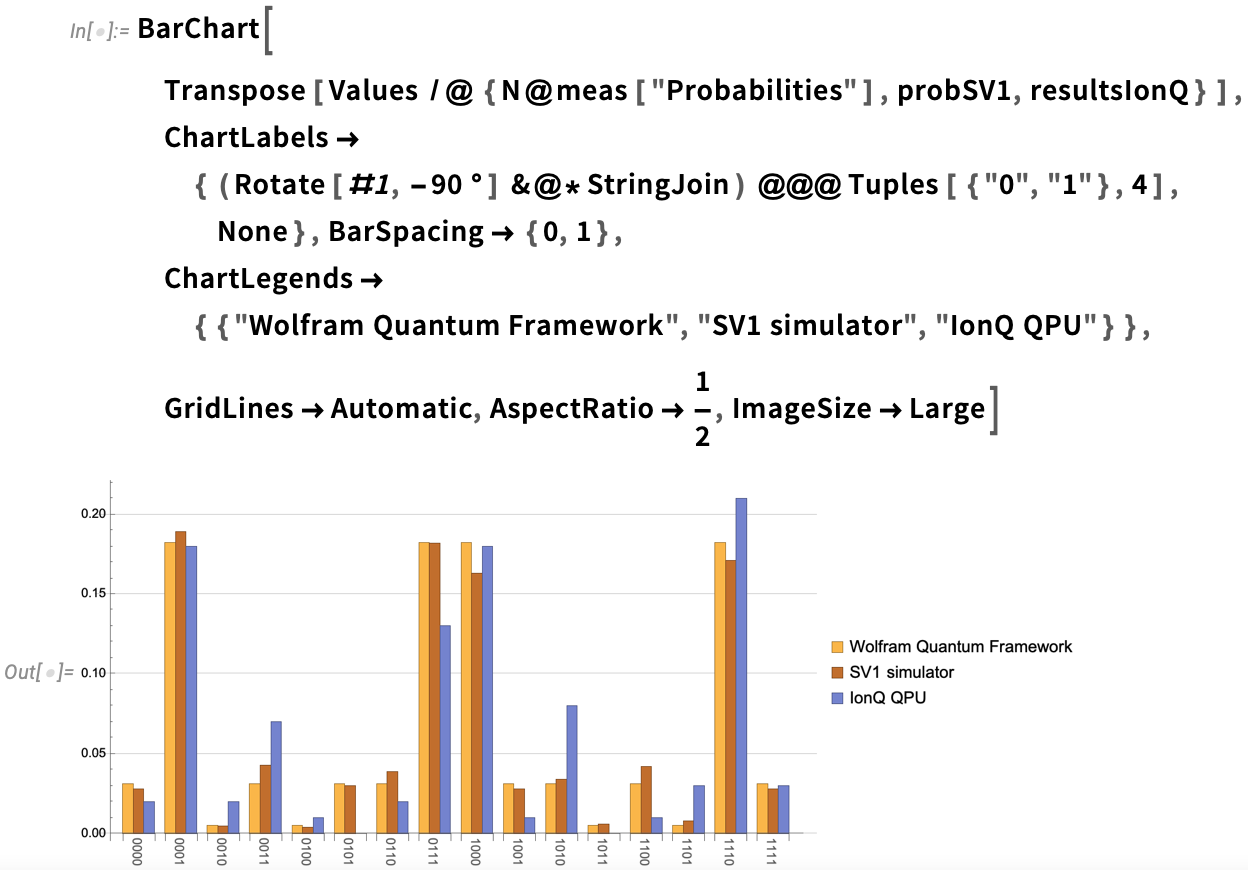Wolfram Language’s quantum functionalities and knowledgebase combined have allowed for the creation of a powerful tool for performing quantum tasks. Together with the advanced functions from Amazon Braket, users are now able to scale their quantum applications and tackle complex computational problems with ease. Researchers, scientists and those hoping to enter the world of quantum technologies can now explore new frontiers and push the boundaries of what is possible with computing methods.

 Learn more about the Wolfram Quantum Framework with streamlined computation for quantum circuits and other finite-dimensional quantum systems.